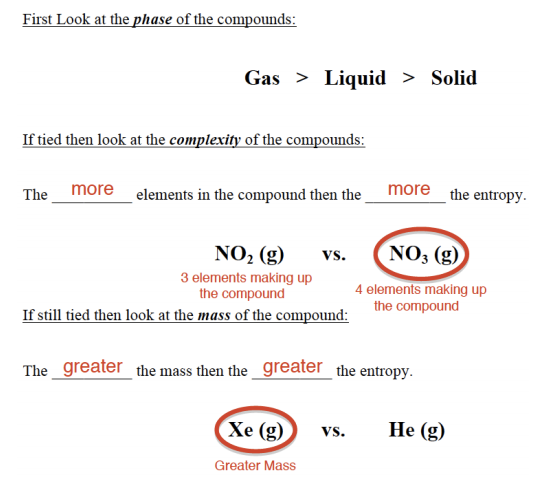# Problem: Arrange the following substances in the order of increasing entropy at 25° C.               CO 2 (s)         Kr (g)         S  8 (s)         O 3 (g)         H 2O (l)         H 2S (l)

###### FREE Expert Solution
91% (166 ratings)
###### Problem Details

Arrange the following substances in the order of increasing entropy at 25° C.

CO 2 (s)         Kr (g)         S  8 (s)         O 3 (g)         H 2O (l)         H 2S (l)Frequently Asked Questions

What scientific concept do you need to know in order to solve this problem?

Our tutors have indicated that to solve this problem you will need to apply the Entropy concept. You can view video lessons to learn Entropy. Or if you need more Entropy practice, you can also practice Entropy practice problems.

What is the difficulty of this problem?

Our tutors rated the difficulty ofArrange the following substances in the order of increasing ...as medium difficulty.

How long does this problem take to solve?

Our expert Chemistry tutor, Jules took 2 minutes and 28 seconds to solve this problem. You can follow their steps in the video explanation above.

What professor is this problem relevant for?

Based on our data, we think this problem is relevant for Professor Dixon's class at UCF.